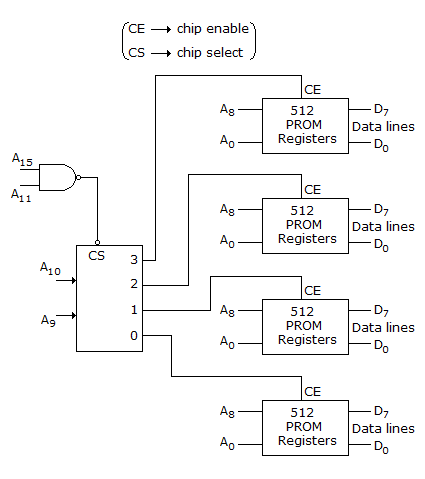# Electronics and Communication Engineering - Exam Questions Papers

26.

Which of the following has a major role in implementation of functions calls in C?

 A. Processor's registers B. Data segment C. The heap D. System stack

Answer: Option D

Explanation:

No answer description available for this question. Let us discuss.

27.

Effective Q of the equivalent electrical circuit of quartz crystal is of the order of

 A. 200 B. 2000 C. 20000 D. 200000

Answer: Option C

Explanation:

No answer description available for this question. Let us discuss.

28.

Two linearly polarized plane waves A and B travelling in free space in the positive x direction meet at a point. Wave A is polarized in the y direction and the wave B is polarized in the z direction. If the two waves are of equal amplitude and are in phase quadrature, the resultant wave will be

 A. circularly polarized B. elliptically polarized C. linearly polarized D. vertically polarized

Answer: Option A

Explanation:

No answer description available for this question. Let us discuss.

29.

Consider the following statements regarding an antenna:

1. It is a transducer
2. Its performance is essentially frequency sensitive
3. It is a reciprocal device
4. With increase in its effective aperture area, width of the radiated beam increases.
Which of the above statements are correct?

 A. 1, 2 and 3 B. 1, 2 and 4 C. 2 and 3 only D. 1 and 4 only

Answer: Option A

Explanation:

No answer description available for this question. Let us discuss.

30.

The range of memory location in given memory interfacing scheme isA. 0000H - 07FFH B. F800H - FFFFH C. 0000H - 03FFH D. 0000H - 3FFFH

Answer: Option B

Explanation:

No answer description available for this question. Let us discuss.

#### Current Affairs 2021

Interview Questions and Answers